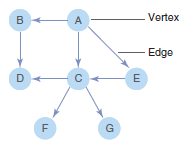# 1.0 - 8.00 - Introduction to Graph Analysis Functions - Teradata Vantage

## Teradata® Vantage Machine Learning Engine Analytic Function Reference

Product
Release Number
1.0
8.00
Release Date
May 2019
Content Type
Programming Reference
Publication ID
B700-4003-098K
Language
English (United States)

Typical uses of graph analysis functions are analysis of social and communications networks and fraud detection. Applications that use these functions include:

• Finding shortest paths
• Computing importance/influence scores
• Predicting unobserved variables based on knowledge of observed variables and network structures

The graph analysis functions use the Teradata SQL-GR™ (SQL-GR) framework. SQL-GR is based on a simple directed-graph data model, where each directed edge is represented as an ordered pair of vertices. To represent an undirected graph, use pairs of directed edges.

## Graphs

A graph is a representation of interconnected objects. An object is represented as a vertex (also called a node)—for example, cities, computers, and people. A link connecting two vertices is called an edge. Edges can represent roads that connect cities, computer network cables, interpersonal connections (such as co-worker relationships), and so on.

Graph ExampleMost ML Engine Graph functions represent a graph with two tables:
• Vertices table
• Edges table

The following two tables represent the graph in the preceding figure.

In the following table, each row represents a vertex.

Vertex Table Example
Vertex City Name
A Albany
B Berkeley
C Cerrito
D Danville
E East Palo Alto
F Foster City
G Gilroy

In the following table, each row represents an edge.

Edges Table Example
Source Destination
A B
A C
A E
B D
C D
C F
C G
E C

## Iterations

When running graph functions, the SQL-GR iteration number in the log might differ from the function iteration number. For example, when running the EigenVectorCentrality function, each EigenVectorCentrality iteration (EI) consumes 2 SQL-GR iterations (GI). For directed graphs, including the overhead, GI = 2*EI +1. For undirected graphs, GI = 2 * EI + 3.

For the PageRank function, the number of SQL-GR iterations equals the number of Pagerank iterations + 1.